# 04/05: #

## Negative Feedback (NFB) #

• The main idea is ‘how do we get an op-amp to output a voltage that isn’t railing?’
• Negative feedback refers to the idea that there is some output amount that is the ideal or intended amount
• If the actual amount becomes larger than this reference, then the system must detect this deviation and bring it down to the reference.
• Similarly, if the actual amount drops too low, the system must bring it back up to the reference.
• Negative-feedback amplifiers helps maintain the output voltage at a constant level despite the fact that the op-amp wants to rail the output.

$$V_{out} = A(U_+ − U_−)$$ $$V_{out} = A(V_{in} − V_{out})$$ $$V_{out} + A \cdot V_{out} = A \cdot V_{in}$$ $$V_{out}(1 + A) = A \cdot V_{in}$$ $$V_{out} = V_{in} \frac{A}{A+1}$$ $$V_{out} \approx V_{in} \text{ for } A \approx \infty$$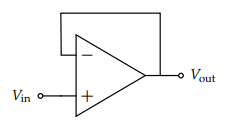To incorporate negative feedback into our op-amp design, we must create a connection that goes from the output into the negative input

• So when $V_{in} \in [V_{SS}, V_{DD}]$ we get a non-clipped output
• Therefore, it’s common to omit the supply terminals when the op-amp is in negative feedback
• When the op-amp is acting as a comparator (i.e. not in negative feedback) the output voltage is basically always either $V_{DD}$ or $V_{SS}$
• However, when an op-amp is in negative feedback, the output voltage is generally independent of the supply
• We are implicitly stating that the supply voltages are large enough that we never clip
• That is, when negative feedback is applied around an amplifier with high open-loop gain it reduces the overall gain of the complete circuit to a desired value due to the loop gain

### Checking for NFB #

One convenient method is to check what happens if the output voltage happens to fluctuate by a little bit above the desired output; when this change occurs and propagates back to the input of the op-amp, does it cause the output to come back down to the desired level? If so, the system is in negative feedback.

1. Zero out all independent sources as in Thevenin-Norton Equivalencies
• Voltage sources become wires and current sources become open circuits
2. “Dink” the output
• If in NFB and $V_{out} \uparrow$, then $U_- \uparrow$ and $U_+ - U_- \downarrow$ (the difference between the higher and lower voltages decreases if the lower voltage increases.)
• Then, $A(U_+ − U_−) \downarrow$ also. From this, we can directly say that $V_{out} \downarrow$
• That is, an increase in the output voltage ultimately led to a decrease in the output voltage.

## Golden Rules #

### 1: $i_- = i_+ = 0$ #

No current flows in/out of the input terminals $U_-$ and $U_+$

• For ideal op-amps, regardless of NFB
• Thus, the behavior of the input terminal’s circuits shouldn’t change

### 2: $U_- = U_+ \iff$ NFB #

• That is, the voltage potential at the positive input terminal (relative to ground) and the voltage at the negative input terminal (relative to the same ground) are the same
• Intuitively, the “error signal” going into the op amp must be zero
• Implied by $A \to \infty$, full proof on page 7

$$v_{in} - f v_{out} = v_c$$ $$v_{out} = A v_c$$ $$\Longrightarrow v_{out} = \frac{A}{1+Af}v_{in}$$

Thus, $v_{in} = 0V \implies v_c = 0V$ and $v_{out}$ can be non-zero even when $v_c = 0$ given $A = \infty$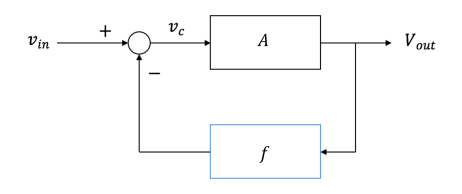## DAC #

Digital-to-Analog Converter: Component that takes in digital signals (bits) and translates them into output analog voltages.

## Signals vs. Supply Voltages #

• In real systems, voltages are typically fluctuating signals
• e.x. changes in light intensity or electrical brain activity read from an EEG
• Signals are voltages, they are different from the supply voltages of an op-amp because they are typically much smaller and they change over time

To distinguish, we will use the following symbol to represent time-varying voltage signals: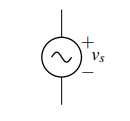• Note that while we are alright having signals as our inputs to op-amps, voltage signals generally should not be used for power since they’d create time-varying behavior

Loading: an electrical component or portion of a circuit that consumes (active) electric power

• The loading effect makes it hard to design circuits because they mean that components will behave differently depending on what they’re connected to
• Specifically, if the circuit afterwards draws current through some element’s $R_{el}$, then the output voltage drops.
• Furthermore, the amount it drops also depends on the rest of the circuit’s resistance $R_{th}$ – but we don’t want to have to redesign our circuit every time we change $R_{el}$

### Example #

• In the real world, the battery (voltage source) has some parasitic, internal resistance along with the wires – $r$
• Thus, the voltage drop across the bulb depends on the value of $r$, which is determined by the battery’s internal structure
• Current flows in this circuit, so some of the battery’s voltage is lost to the internal resistance of the battery
• Most of the time this just means the battery/wires heat up

$$V_{lightbulb} = \frac{R_l}{R_l + r} V_{battery}$$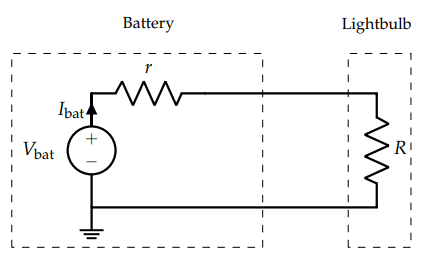• We want some way to ensure that no matter what $r$ is, the voltage dropped across the bulb was a constant, isolating it from the source

## Buffering #

Buffer (amplifier): provides electrical impedance transformation from one circuit to another, with the aim of preventing the signal source from being affected by whatever currents (or voltages, for a current buffer) that the load may be produced with

• Buffers are a powerful tool because they allow us to split circuits into blocks that we can analyze separately and then combine later
• When circuit blocks behave the same way regardless of what they’re are connected to, we don’t need to worry about what’s inside, making it much easier to design complex circuits.

### Example #

• We can throw a unity gain buffer in our situation above to solve our issue
• In a unity gain buffer, $v_{in} = v_{out}$
• The voltage at the non-inverting input is $V_{bat}$ since no current can flow into the op-amp ( GR#1) and the same voltage appears at the output because of our unity-gain buffer
• This is the case if, by Ohm’s law, current must flow out of the op-amp– which is perfectly fine since it’s only the inputs that current cannot flow into or out of.

$$V_{lightbulb} = U_{out} = V_{battery}$$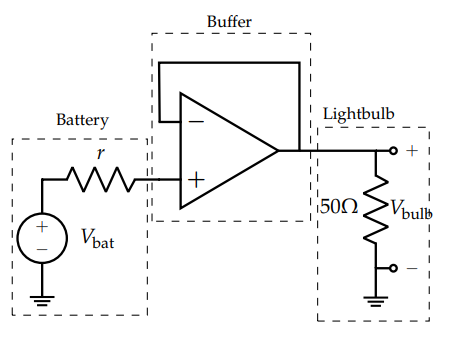• Now the full $V_{bat}$ will be dropped across the bulb, and we don’t need to worry about any loading effects
• No matter what the $r$ or $R$ values are, the circuit’s behavior is consistent

## Design Procedure #

### 1: Specification #

Concretely restate the goals for the design.

• Frequently, a design prompt will include a lot of text, so we’d like to restate all of the most important features of our design. We’ll refer to these specifications later to determine if our design is complete.

### 2: Strategy #

Describe your strategy (often in the form of a block diagram) to achieve your goal.

• To do this, start by thinking about what you can measure vs. what you want to know
• For example in our capacitive touchscreen, we want to know if there is a touch and we can measure voltage.
• Since we know that a touch can change the capacitance, we break this down into the following block diagram: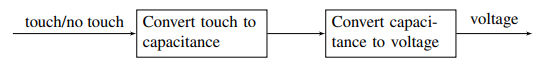### 3: Implementation #

Implement the components described in your strategy.

• This is where pattern matching is useful: remind yourself of blocks you know, (ex. voltage divider, inverting amplifier) and check if any of these can be used to implement steps of your strategy.

### 4: Verification #

Check that the design from 3 does what we specified in 1

• Check block-to-block connections, as these are the most common point for problems.
• Does one block load another block causing it to behave differently than expected?
• Are there any contradictions (ex. a voltage source with both ends connected by a wire, or a current source directed into an open circuit)?